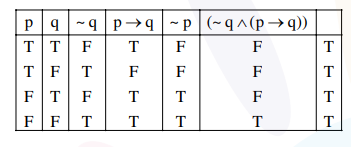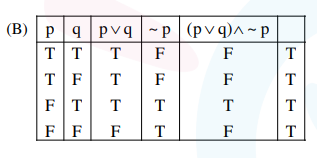# Solve this following

Question:

For the statements $\mathrm{p}$ and $\mathrm{q}$, consider the following compound statements :

(a) $(\sim \mathrm{q} \wedge(\mathrm{p} \rightarrow \mathrm{q})) \rightarrow \sim \mathrm{p}$

(b) $((\mathrm{p} \vee \mathrm{q}) \wedge \sim \mathrm{p}) \rightarrow \mathrm{q}$

Then which of the following statements is correct?

1. (a) and (b) both are not tautologies.

2. (a) and (b) both are tautologies.

3. (a) is a tautology but not (b).

4. (b) is a tautology but not (a).

Correct Option: , 2

Solution:

(A)Both are tautologies